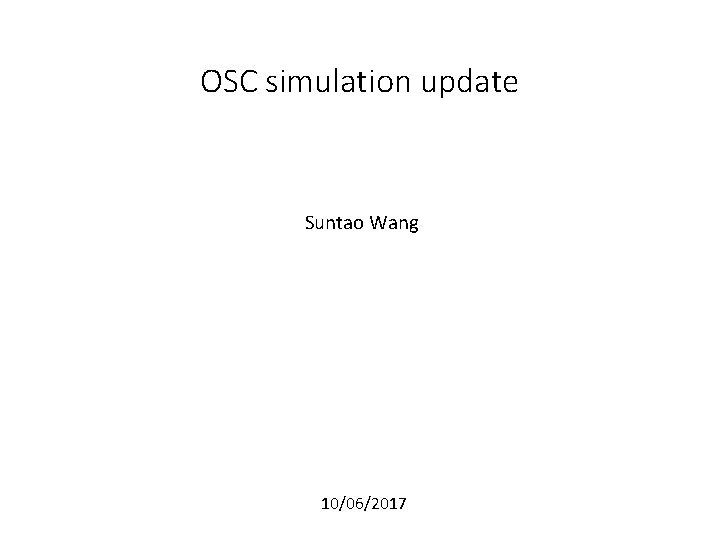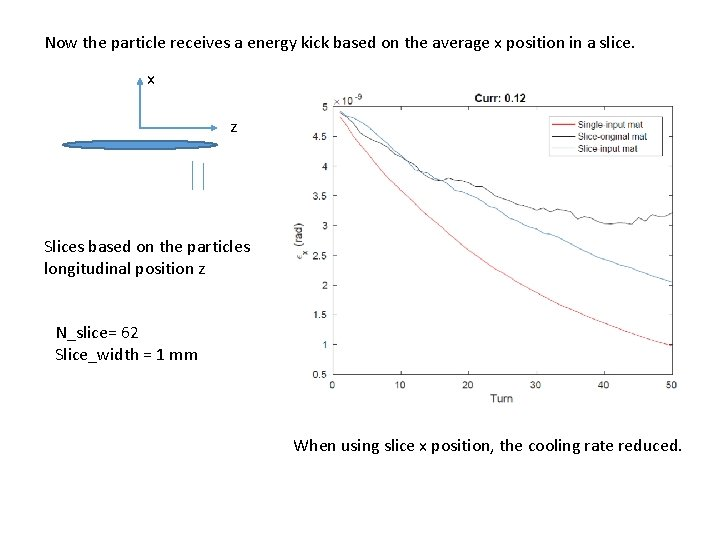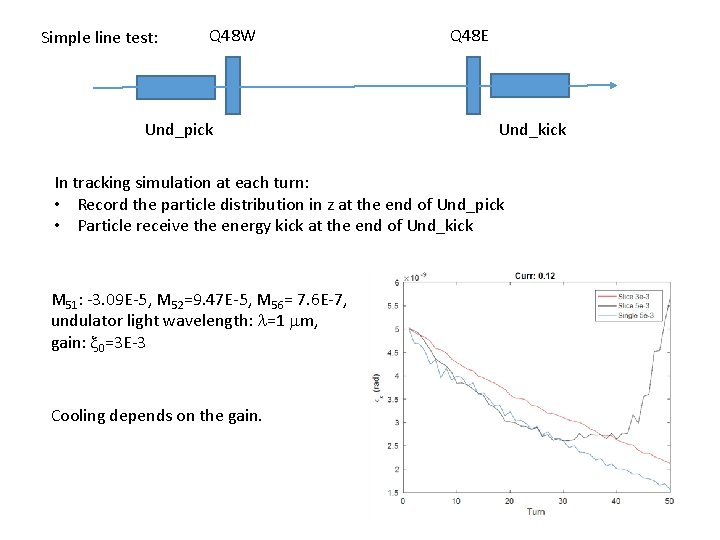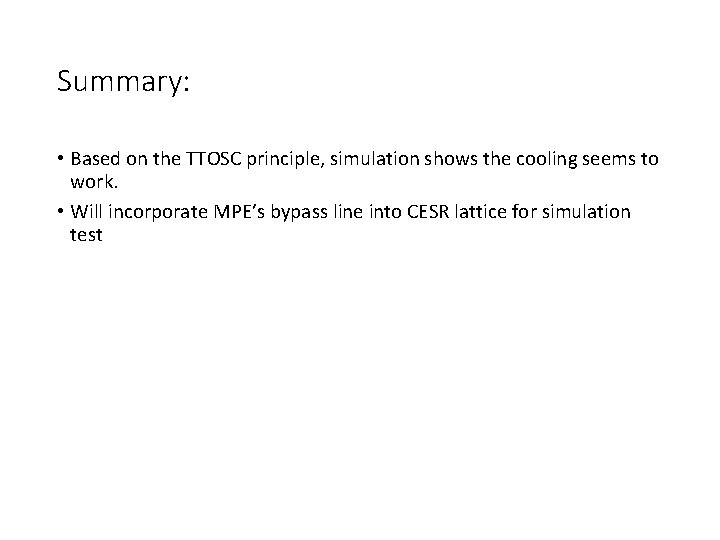# OSC simulation update Suntao Wang 10062017 Transittime optical

• Slides: 7OSC simulation update Suntao Wang 10/06/2017Transit-time optical stochastic cooling (TTOSC) Particle receives the energy kick dp/p from the kicker undulator: where x 0 is the amplitude, k=2 p/l, M 5 n are the elements of the 6 x 6 transfer matrix from the pickup undulator to the kicker undulator, (x, qx, Dp/p) are the particle horizontal coordinate, angle, and relative momentum deviation in the pickup. g: Optical amplifier gain q: electron charge e 0: EM wave amplitude of the light from the pickup Nu: number of kicker undulator periods lu: the period length of the kicker undulator K: the pickup undulator K parameter Eb: electron beam energy g: 948 @ 500 Me. V In simulation, x 0 is one parameterSingle element test: 100 particles, track for 50 turns, excitation and damping turned on Normal emittance are extracted from the sigma matrix every turn Q 45 E: large horizontal dispersion 2. 05 m in the test lattice (2. 1 Ge. V Cesr. TA lattice) M 51: -1. 35 E-7, M 52=1. 26 E-6, M 56= 3 E-8, undulator light wavelength: l=1 mm, gain: x 0=1 E-2 Each particle receives the energy kick based on its own (x, x’, z’) Only horizontal emittance reduced. The energy spread and bunch length slight increased.Single element test: 100 particles, track for 50 turns, excitation and damping turned on Q 45 E: large horizontal dispersion 2. 05 m in the test lattice (2. 1 Ge. V Cesr. TA lattice) M 51: -1. 35 E-7, M 52=1. 26 E-6, M 56= 1 E-6, undulator light wavelength: l=1 mm, gain: x 0=1 E-2 Each particle receives the energy kick based on its own (x, x’, z’) Both horizontal and longitudinal emittance reduced. The energy spread reduced either.Now the particle receives a energy kick based on the average x position in a slice. x z Slices based on the particles longitudinal position z N_slice= 62 Slice_width = 1 mm When using slice x position, the cooling rate reduced.Simple line test: Q 48 W Und_pick Q 48 E Und_kick In tracking simulation at each turn: • Record the particle distribution in z at the end of Und_pick • Particle receive the energy kick at the end of Und_kick M 51: -3. 09 E-5, M 52=9. 47 E-5, M 56= 7. 6 E-7, undulator light wavelength: l=1 mm, gain: x 0=3 E-3 Cooling depends on the gain.Summary: • Based on the TTOSC principle, simulation shows the cooling seems to work. • Will incorporate MPE’s bypass line into CESR lattice for simulation test